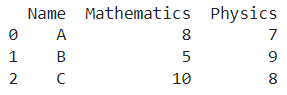# Python Pandas Get Index Of Rows Which Column Matches Certain Value?

A DataFrame is the primary data structure of the Pandas library in Python and is commonly used for storing and working with tabular data.

A common operation that could be performed on such data is to get the index of the rows where a column entry matches a certain value in order to extract more information from it.

To start working with Pandas, we first need to import it:

### Python 3 Code :

``import pandas as pd``

## Running Example

Let us understand this operation with the help of an example. Consider the following DataFrame containing 3 students with names A, B, and C and their corresponding marks (out of 10) for two subjects, Mathematics and Physics.Code snippet for generating the above DataFrame :

### Python 3 Code :

``````import pandas as pd

# Dictionary for our data
data = {'Name' : ['A', 'B', 'C'], 'Mathematics' : [8, 5, 10], 'Physics' : [7, 9, 8]}

# DataFrame for the dictionary
df = pd.DataFrame(data)

# Printing the DataFrame
print(df)``````

Here, data is a dictionary we created to initialize the DataFrame. For this, we use the DataFrame() function of the Pandas library which takes the dictionary as an argument and returns the required DataFrame.

Now, let’s say we need to get the index of the rows where the corresponding students have 8 marks in Physics.

Since the student with the name as C meets this condition, the corresponding row index, that is 2, would be the desired result. Let us say we want to get this result in the form of a list.

Remember that indices in python are zero-based. The resulting output would look like this :



Let us look at different ways of performing this operation on a given DataFrame :

## Using the DataFrame.index property to get the index of the row in pandas

In this method, we use the DataFrame.index property to get the index of the rows where a column entry matches a certain value.

Here we want the index of the corresponding row of the students that have exactly 8 marks in physics. We do this by using the equivalence condition as a parameter for the dataframe.index property.

This condition here becomes df[‘Physics’] == 8. This dataframe.index property returns an index object.

We use the .tolist() function on the returned object to having a nice output in the form of a list which is stored in a variable named idx.

``````# Importing pandas
import pandas as pd

# Dictionary for our data
data = {'Name' : ['A', 'B', 'C'], 'Mathematics' : [8, 5, 10], 'Physics' : [7, 9, 8]}

# DataFrame for the dictionary
df = pd.DataFrame(data)

# Performing the operation
idx = df.index[df['Physics'] == 8].tolist()

# Printing
print(idx)``````

Output :



Instead of using df[‘Physics’] to access the physics column of the DataFrame as shown earlier, we can also use df.Physics to access it and get the same results.

Let us take a look at the corresponding code snippet and generated output for this method :

``````# Importing pandas
import pandas as pd

# Dictionary for our data
data = {'Name' : ['A', 'B', 'C'], 'Mathematics' : [8, 5, 10], 'Physics' : [7, 9, 8]}

# DataFrame for the dictionary
df = pd.DataFrame(data)

# Performing the operation
idx = df.index[df.Physics == 8].tolist()

# Printing
print(idx)``````

Output :



## Using the Numpy.where() function to get the index of the row in pandas

To use NumPy, we need to first import it in a similar manner as we did for pandas. The code for this is as follows -

``import numpy as np``

In this method, we use the numpy.where() function to get the index of the rows where a column entry matches a certain value.

Here, we want the index of the corresponding row of the students that have exactly 8 marks in physics. We do this by using the equivalence condition as a parameter for the numpy.where() function. This condition here becomes df[‘Physics’] == 8.

This numpy.where() function returns a python tuple containing the desired indices. We use the in-built python list() function along with the returned object as a parameter and access its first and only element to have a nice output in the form of a list which is stored in a variable named idx.

``````# Importing pandas and numpy
import pandas as pd
import numpy as np

# Dictionary for our data
data = {'Name' : ['A', 'B', 'C'], 'Mathematics' : [8, 5, 10], 'Physics' : [7, 9, 8]}

# DataFrame for the dictionary
df = pd.DataFrame(data)

# Performing the operation
idx = list(np.where(df['Physics'] == 8))

# Printing
print(idx)``````

Output :



Instead of using df[‘Physics’] to access the physics column of the DataFrame as shown earlier, we can also use df.Physics to access it and get the same results. Let us take a look at the corresponding code snippet and generated output for this method :

``````# Importing pandas and numpy
import pandas as pd
import numpy as np

# Dictionary for our data
data = {'Name' : ['A', 'B', 'C'], 'Mathematics' : [8, 5, 10], 'Physics' : [7, 9, 8]}

# DataFrame for the dictionary
df = pd.DataFrame(data)

# Performing the operation
idx = list(np.where(df.Physics == 8))

# Printing
print(idx)``````

Output :



## Conclusion

In this topic, we have learned to delete rows from an existing Pandas DataFrame based on certain conditions, following a running example of test scores of students in different subjects, thus giving us an intuition of how this concept could be applied in real-world situations. Feel free to reach out to info.javaexercise@gmail.com in case of any suggestions.

Trending